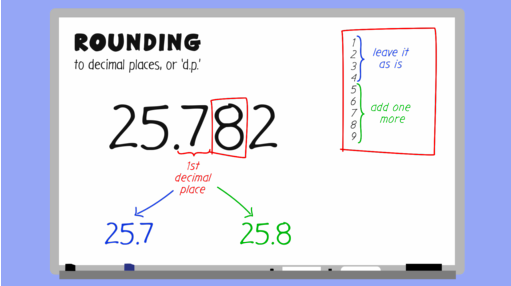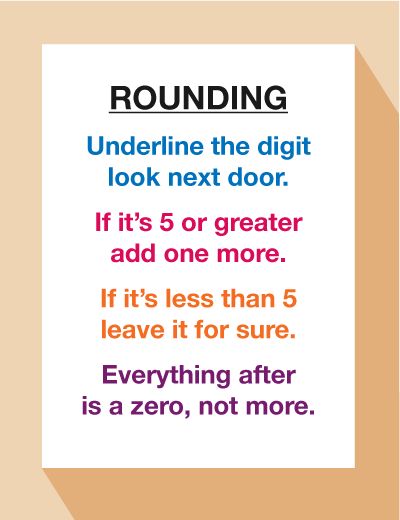Everyday maths 2 (Wales)

Start this free course now. Just create an account and sign in. Enrol and complete the course for a free statement of participation or digital badge if available.

Free course

# 3.1 Rounding to a degree of accuracy

Watch the short video below to see an example of how to round to 1, 2 and 3 decimal places.Skip transcript

#### Transcript

Rounding can be used when you're asked to give an answer to a given degree of accuracy. This is called rounding to decimal places, or d.p. In this video, you'll look at how to round to one, two, and three decimal places.

Take the number 25.782. How would you write this rounded to one decimal place? This is the first decimal place. Rounded to 1 d.p., the number could be either 25.7 or 25.8. Look at the digit next to the number 7. If this number is equal to or greater than 5, add one more. If it's less than 5, leave it. In this case, 8 is greater than 5, so our number rounded to 1 d.p. is 25.8.

Now, let's around a number to two decimal places. A common example of when you would do this in daily life is when dealing with money. If you were at a restaurant and needed to split a bill of £87.95 between three people, you would first calculate the division. £87.95 divided by 3 equals £29.3166667. Clearly, you cannot pay this exact amount. So how much would you pay if the amount per person was rounded to two decimal places?

This is the second decimal place. Look at the number next to it. Is it greater than or equal to 5? 6 is more than 5, so you need to add 1. The amount to pay is £29, 32 pence.

Let's try another example. How would you around the number 35.496 to two decimal places? This is the second decimal place. Look at the number next to it. 6 is greater than 5, so you need to add one more. In this case, the rounded number would be 35.50.

Can you round to three decimal places? Have a go with this number: 412.5762. The number next to the third decimal place is 2, which is less than 5. This means the correctly rounded number is 412.576.

End transcript

Interactive feature not available in single page view (see it in standard view).Figure 5 A rounding rhyme

## Activity 8: Rounding skills

Practise your rounding skills by completing the below.

1. What is 24.638 rounded to one decimal place?

2. What is 13.4752 rounded to two decimal places?

3. What is 203.5832 rounded to two decimal places?

4. What is 345.6794 rounded to three decimal places?

5. What is 3.65 rounded to the nearest whole number?

6. What is £199.755 to the nearest penny?

7. What is £37.865 to the nearest pound?

8. What is 61.607 kg to the nearest kg?

1. 24.6

2. 13.48

3. 203.58

4. 345.679

5. 4

6. £199.76

7. £38

8. 62 kg

FSM_2_CYMRU